• ## MATLAB截取图像特定区域

万次阅读 多人点赞 2019-04-14 23:22:16
MATLAB截取图像特定区域 你好！你可以仔细阅读这篇文章，了解一下MATLAB的截取图像特定区域的方法。 二话不说先上代码 代码片. save_path='D:\picture\'; img_path_list = dir(strcat(save_path,'*....
MATLAB截取图像特定区域
你好！你可以仔细阅读这篇文章，了解一下MATLAB的截取图像的特定区域的方法。
截取程序
imcrop函数 功能：用于返回图像的一个裁剪区域。可把图像显示在一个图像窗口中。 代码片示例.
 picture_1 =imcrop(picture,[x(1),y(1),abs(x(1)-x(2)),abs(y(1)-y(2))])   %切割图像，起始坐标点（x1,y1）截取到终止坐标点(x2,y2)

代码说明：picture为要裁剪的图片 picture_1裁剪后的图片 x(1),y(1)裁剪起始坐标点 abs(x(1)-x(2)),abs(y(1)-y(2))裁剪长度
读取图片
save_path='D:\picture\';     %图片地址文件夹
img_path_list = dir(strcat(save_path,'*.jpg'));    %仅读取文件夹中的.jpg文件
img_num=length(img_path_list);  %判断图片个数
for i = 1:img_num        %采用循环方式读取文件
picture_name =img_path_list(i).name;
%imshow(picture);
end

获取截取点坐标
ginput函数
功能: 允许用户以交互方式使用鼠标选定要剪切的区域以此获取两个坐标点并以矩形方式裁剪
[x,y] = ginput(n)   %n为你想选的点的个数，鼠标点的点的横坐标和纵坐标就会保存到[x,y]中。然后再输出x,y便可获取在图片上需要截取的点的坐标！

完整代码
代码片.
save_path='D:\picture\';

img_path_list = dir(strcat(save_path,'*.jpg'));

img_num=length(img_path_list);   %判断图片个数

for i = 1:img_num     %因为拍照片的时候固定好了位置所以用一个for循环就可以截取出所有的图片的数字

picture_name =img_path_list(i).name;

imshow(picture);

[x,y]=ginput(2);   %先用的ginput函数获取图片中数字的起始坐标

%x=[1.0e+03 *0.7145,1.0e+03 *1.0305];

%y=[234.5000,670.5000];

picture_1 =imcrop(picture,[x(1),y(1),abs(x(1)-x(2)),abs(y(1)-y(2))]);  %切割图像，起始坐标点（x1,y1）截取到终止坐标点(x2,y2)

imwrite(picture_1,[num2str(i),'.jpg']);%将图片保存在程序所在文件夹中

end


截取前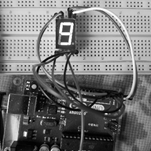截取后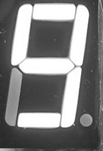展开全文• 比如选取一个不规则四边形内的点: 确定四个点的坐标, declaim四边形: xv=[x1,x2,x3,x4,x1]; % sym2double yv=[y1,y2,y3,y4,y1]; figure plot(xv,yv) xlim([0,2.4]) ylim([-2.4,0]); 2. 定义坐标区域内的点: ...
比如选取一个不规则四边形内的点:
确定四个点的坐标, declaim四边形:
xv=[x1,x2,x3,x4,x1]; % sym2double
yv=[y1,y2,y3,y4,y1];

figure
plot(xv,yv)
xlim([0,2.4])
ylim([-2.4,0]);2. 定义坐标区域内的点:
xVector=0:0.05:2.4;
yVector=-2.4:0.05:0;

[x,y]=meshgrid(xVector,yVector); % obtain all coordinates

xx=reshape(x,1,numel(x));
yy=reshape(y,1,numel(y));

使用inpolygon来判断是否在特定区域内
in=inpolygon(x,y,xv,yv);

figure
plot(xv,yv,x(in),y(in),'.r',x(~in),y(~in),'.b') % red is in area, blue out4. 获取区域内的坐标:
inX=x(in);
inY=y(in);

另外参考: matlab之如何将矩阵特定位置的元素置零?
展开全文• 关键是一个叫做roipoly的函数，它的输入是一副图像，然后在图像窗口中手动划定一个区域，然后输出一个黑白图像，其中你标记的地方为白，其余地方为黑。下面这个程序实现了如何从一个大图中手动的拿出一小部分。 ...

关键是一个叫做roipoly的函数，它的输入是一副图像，然后在图像窗口中手动划定一个区域，然后输出一个黑白图像，其中你标记的地方为白，其余地方为黑。下面这个程序实现了如何从一个大图中手动的拿出一小部分。

function g=getROIbyInter(f)

f=rgb2gray(f);
bw=roipoly(f);
[I,J]=find(bw==1);
g=f(min(I):max(I),min(J):max(J));

end

结果：
输入：输出：转载于:https://www.cnblogs.com/naniJser/archive/2012/12/12/2815466.html
展开全文• 下面来介绍一下如何在MATLAB中对图像的某些特定像素值进行处理，具体如下： 1、打开MATLAB，在其主界面的编辑器中写入下列代码： B=imread('eight.tif'); %读取图像 g=[222 272 300 270 221 194]; %选取像素区间...
下面来介绍一下如何在MATLAB中对图像的某些特定像素值进行处理，具体如下：
1、打开MATLAB，在其主界面的编辑器中写入下列代码：
B=imread('eight.tif');      %读取图像
g=[222 272 300 270 221 194]; %选取像素区间块
f=[21 21 75 121 121 75];     %选取像素区间块
j=roifill(B,g,f);           %特定区域掩盖
figure,imshow(B);
figure,imshow(j)

2、保存代码至自定义路径下，点击运行，结果如下：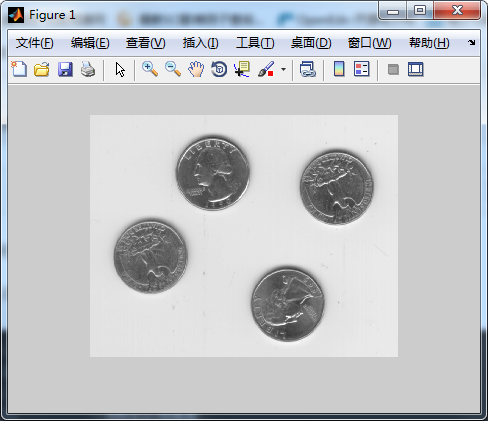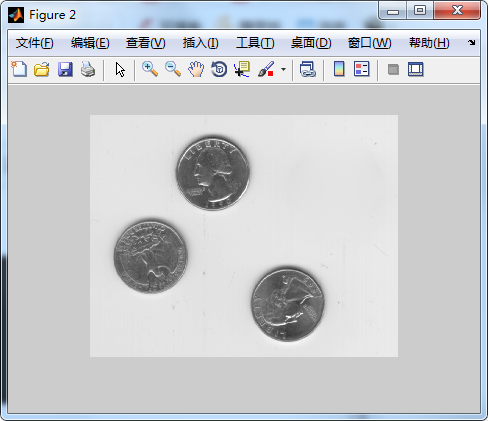如图所示，可以明显看出，处理后的图像中只显示了三枚硬币，这就是把之前第四枚硬币的像素值区域掩盖的结果，也可以根据用户自己的需求进行掩盖和虚化。
3、新建一个编辑文本，写入下列代码：
B=imread('eight.tif');       %加载图片
g=[222 272 300 270 221 194]; %像素区域选择1
f=[21 21 75 121 121 75];     %像素区域选择2
BW=roipoly(B,g,f);
h=fspecial('unsharp');
j=roifilt2(h,B,BW);
figure,imshow(B);
figure,imshow(j)
4、保存该代码至自定义路径下，点击运行，结果如下：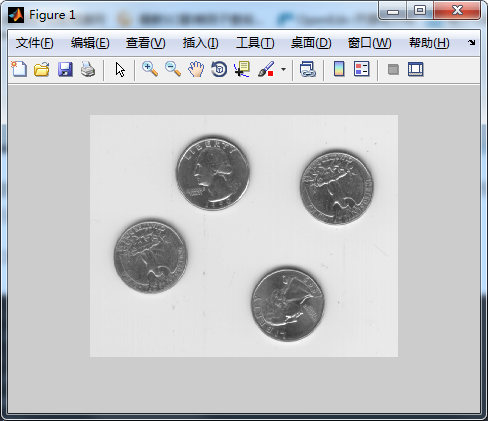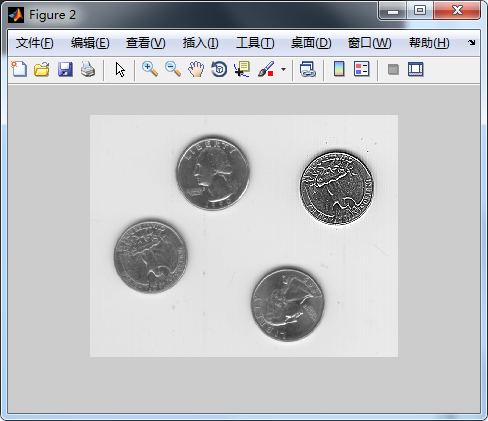如图所示，可以明显看出，上诉代码的结果就是对原图的第四个硬币进行图像增强，效果很好。
至此基本介绍完毕，请大家继续关注！！！
展开全文• [求助]在图像选取区域，将区域变成黑色 最近碰到一个难题，是这样的：导师要处理一个2D图像， 因为要交互，需要一个小界面，我弄了一个GUI，在GUI中显示图像，有一块不需要处理，因此，需要将这一块涂黑，可以在图...
• %图片路径名称 a为m*n*3矩阵 m，n分别为宽高 3表示RGB分量 dd1=(a(:,:,1)=240&a(:,:,2)=205&a(:,:,3)=30); %由RGB颜色范围抠图 结果为逻辑矩阵（只包含0与1） [m,n]=size(dd1); z=zeros(m,n); image(cat(3,dd1,z,z))
•  在统计过程中，需要对特定的对象进行数据处理与分析，本文为图像处理数据分析的过程中提取感兴趣的区域，并进行保存的MATLAB程序，有需要的可以参考。  本文根据统计目标区域与背景区域的特征这个应用写的。 ...
• ## ROI区域选取，Matlab

千次阅读 2020-03-19 17:15:31
一幅图像，我们需要处理的区域可能只占整幅图像的一小部分，通过鼠标进行手动选取，展示图像，并且获得ROI图像的起始行列坐标。...%选取图像的ROI区域----------------------------------- figur...计算机视觉
• Mat 类提供了多种方便的方法来选择图像的局部区域。使用这些方法时需要注意,这些方法并不进行内存的复制操作。如果将局部区域赋值给新的 Mat 对象,新对象与原始对象共用相同的数据区域,不新申请内存,因此这些方法的...Mat::row
• 选取图像局部区域 Mat 类提供了多种方便的方法来选择图像的局部区域。 使用这些方法时需要注意，这些方法并不进行内存的复制操作。如果将局部区域赋值给新的 Mat 对象，新对象与原始对象共用相同的数据区域，不新...opencv
• 转自：https://blog.csdn.net/shenwanjiang111/article/details/54318958Mat 类提供了多种方便的方法来选择图像的局部区域。使用这些方法时需要注意,这些方法并不进行内存的复制操作。如果将局部区域赋值给新的 Mat ...opencv
• 二是从人眼得视觉特征出发，通过模拟人眼得视觉特点，寻找特定得视觉敏感区域，并将这些视觉敏感区域排序作为ROI。 本文介绍差影法（对图像进行代数运算得一种不同的叫法）、交互式提取法、自动图像分割提取法。 差...
• 选择你感兴趣的区域，截取图片，然后下一步对你感兴趣的区域继续进行操作
• %第一步：从图片选取矩形框区域 I = imread('o.png'); [A,rect] = imcrop(I); imshow(A); rect %第二步：根据rect确定：在原图中绘制的矩形的坐标，注意rect的格式[m n l k]->[(m,n) (m+l,n+k)]->[(n,m) (n+k...
• 导师给了一个任务，主要是在一张图片中识别出特定图像，并标记出相应物体的位置（在坐标图上呈现），分别用c语言和matlab都实现一下来练手。 思路 参考了一些网上的代码，我这次实现的主要思路就是用鼠标获取目标...opencv c语言python opencv 计算机视觉 人工智能
• ## matlab特定颜色提取

万次阅读 多人点赞 2019-02-28 10:02:33
matlab特定颜色提取 一、理论基础 在电脑中，RGB的所谓“多少”就是指亮度，并使用整数来表示。通常情况下，RGB各有256级亮度，用数字表示为从0、1、2…直到255。注意虽然数字最高是255，但0也是数值之一，因此共256...颜色提取
• %鼠标选中特定区域（支持单个区域选取） clc;clear all;close all; load matlab.mat I1=I; figure,imagesc(I1); hold on [x,y,flag]=ginput(1); m(1)=x; n(1)=y; k=2; while(flag==1) [x1,y1,flag1]=ginput(1); if...
• ## 6. 特定区域处理

万次阅读 2017-01-04 10:12:57
在进行图像处理时，有时只需要对图像中的某个特定区域进行处理，而并不需要对整个图像进行处理。比如要对用户选定的一个特定的一个区域作均值滤波或对比度增强，MATLAB就可以只对特定的区域进行处理。 1.指定感...
• imBw = im2bw(I); %转换为二值化图像 imLabel = bwlabel(imBw); %对各连通域进行标记 stats = regionprops(imLabel,'Area');... %选取特定像素大小的连通区域 img = ismember(imLabel,index); %获取最大连通域图像shell
• 现有的图像分割方法主要有：基于阈值的分割方法、基于区域的分割方法、基于边缘的分割方法、基于特定理论的分割方法等。 聚类分析是一种无监督的学习方法，能够从研究对象的特征数据中发现关联规则， 因而是一种强大...聚类 算法
• 通过python改变图片特定区域的颜色详解首先让我祭出一张数学王子高斯的照片，这位印在德国马克上的神人有多牛呢? 他是近代数学的奠基人之一，与牛顿, 阿基米德并称顶级三大数学家，随便找一个编程语言的数学库，里面...
• 【实例简介】基于MATLAB彩色图像分割，采用聚类算法，MATLAB工具(1)利用RGB空间到LAB空间转换公式(1)-(9);五· Kmean的聚类算法步骤:(1)读入图像数据,把彩包图像从RGB颜色空间转化到Lab空间。L表示亮度层色度a表示...
• 使用matlab进行交互式图像处理，满足特定区域图像处理
• 第2章 Matlab数字图像处理基础 Matlab是MathWorks公司开发的一款工程数学计算软件。不同于C++、Java、Fortran等高级编程语言是对机器行为进行描述，Matlab 是对数学操作进行更直接的描述。Matlab 图像处理工具箱...
• 毕业设计(论文)任务书_____________________________________________________________一、学生姓名：学号：二、题目:图像分割算法分析及MATLAB编程实现三、题目来源：真实、自拟四、结业方式：设计、论文五、主要...
• 本次设计主要研究了数字图像处理中图像分割中的阈值分割法和区域生长法，此次研究主要是以MATLAB软件为平台，采用了区域生长法编写设计代码程序，实现了数字图像区域特征检测，包括提取了周长、面积和重心坐标，...dwr python 计算机视觉 机器学习 人工智能
• MATLAB图像分割毕业设计开题报告 上 海 工 程 技 术 大 学毕业设计(毕业论文)开题报告学 院 电子电气学院专 业 自动化班级学号 0212082 021206231学 生 於恺律指导教师 陈剑雪题 目 基于支持向量机的数字图像分割...
• 在Windows操作系统下的MATLAB2019b应用程序中编写程序，待程序调试完成后，通过选取相同尺寸、不同质量的三幅图像分别利用变换函数（线性函数、对数函数）和直方图均衡化方法进行灰度变换来探究基于灰度变换的图像...高斯拉普拉斯算子 直方图...

# matlab选取图像特定区域matlab 订阅